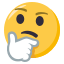## Geometry Marathon : Season 3

For discussing Olympiad level Geometry Problems
Asif Hossain
Posts: 169
Joined: Sat Jan 02, 2021 9:28 pm

### Re: Geometry Marathon : Season 3

Mehrab4226 wrote:
Mon Mar 29, 2021 10:08 pm
Asif Hossain wrote:
Mon Mar 29, 2021 10:01 pm
~Aurn0b~ wrote:
Sun Mar 28, 2021 11:21 pm
$\textbf{Problem 60}$

Denote by $M$ midpoint of side $BC$ in an isosceles triangle $\triangle ABC$ with $AC = AB$. Take a point $X$ on a smaller arc $MA$ of circumcircle of triangle $\triangle ABM$. Denote by $T$ point inside of angle $BMA$ such that $\angle TMX = 90$ and $TX = BX$.

Prove that $\angle MTB - \angle CTM$ does not depend on choice of $X$.
What did you meant by inside of $\angle BMA$??(sry if this sound foolish)
I think he meant $\triangle BMA$
then i think the $TX=BX$ holds iff $T=B$
Hmm..Hammer...Treat everything as nail

Asif Hossain
Posts: 169
Joined: Sat Jan 02, 2021 9:28 pm

### Re: Geometry Marathon : Season 3

~Aurn0b~ wrote:
Mon Mar 29, 2021 10:44 pm
Asif Hossain wrote:
Mon Mar 29, 2021 10:01 pm
~Aurn0b~ wrote:
Sun Mar 28, 2021 11:21 pm
$\textbf{Problem 60}$

Denote by $M$ midpoint of side $BC$ in an isosceles triangle $\triangle ABC$ with $AC = AB$. Take a point $X$ on a smaller arc $MA$ of circumcircle of triangle $\triangle ABM$. Denote by $T$ point inside of angle $BMA$ such that $\angle TMX = 90$ and $TX = BX$.

Prove that $\angle MTB - \angle CTM$ does not depend on choice of $X$.
What did you meant by inside of $\angle BMA$??(sry if this sound foolish)
I think it kinda means TM is inside the angle BMA(That is what i assumed when i tried to solve it, i also never heard anything like it, but that's what the problem states). It's from 2007 IMOSL u can see the question if u want to
It said angle domain....i think it is the fancier of saying $T$ is on the opposite region of $X$ respect to $AM$
Hmm..Hammer...Treat everything as nail

Dustan
Posts: 64
Joined: Mon Aug 17, 2020 10:02 pm

### Re: Geometry Marathon : Season 3

Asif Hossain wrote:
Tue Mar 30, 2021 8:58 am
Mehrab4226 wrote:
Mon Mar 29, 2021 10:08 pm
Asif Hossain wrote:
Mon Mar 29, 2021 10:01 pm

What did you meant by inside of $\angle BMA$??(sry if this sound foolish)
I think he meant $\triangle BMA$
then i think the $TX=BX$ holds iff $T=B$
i don't think soAsif Hossain
Posts: 169
Joined: Sat Jan 02, 2021 9:28 pm

### Re: Geometry Marathon : Season 3

Dustan wrote:
Tue Mar 30, 2021 10:10 am
Asif Hossain wrote:
Tue Mar 30, 2021 8:58 am
Mehrab4226 wrote:
Mon Mar 29, 2021 10:08 pm

I think he meant $\triangle BMA$
then i think the $TX=BX$ holds iff $T=B$
i don't think soI am not sure but it is highly suspicious* because $B$ is kinda the farthest point...at least i couldn't find any.
You may try to prove it Set $M(0,0),B(a,0),A(0,b),T(x',y')$ and $X(m,n)$ where $m$ and $n$ satisfies $(m- \frac{a}{2})^2 +(n- \frac{b}{2})^2 =\frac{\sqrt{a^2+b^2}}{2}$ and $0<n<b$ then noticing $0<x' <a$ and $0<y'<b$
Hmm..Hammer...Treat everything as nail

~Aurn0b~
Posts: 43
Joined: Thu Dec 03, 2020 8:30 pm

### Re: Geometry Marathon : Season 3

$\textbf{Problem 61}$

Let $ABCD$ be a isosceles trapezoid with $AB\parallel CD$ and $\Omega$ is a circle passing through $A,B,C,D$. Let $\omega$ be the circle passing through $C,D$ and intersecting with $CA,CB$ at $A_1$, $B_1$ respectively. $A_2$ and $B_2$ are the points symmetric to $A_1$ and $B_1$ respectively, with respect to the midpoints of $CA$ and $CB$. Prove that the points $A,B,A_2,B_2$ are concyclic.

Asif Hossain
Posts: 169
Joined: Sat Jan 02, 2021 9:28 pm

### Re: Geometry Marathon : Season 3

~Aurn0b~ wrote:
Mon Apr 05, 2021 7:36 pm
$\textbf{Problem 61}$

Let $ABCD$ be a isosceles trapezoid with $AB\parallel CD$ and $\Omega$ is a circle passing through $A,B,C,D$. Let $\omega$ be the circle passing through $C,D$ and intersecting with $CA,CB$ at $A_1$, $B_1$ respectively. $A_2$ and $B_2$ are the points symmetric to $A_1$ and $B_1$ respectively, with respect to the midpoints of $CA$ and $CB$. Prove that the points $A,B,A_2,B_2$ are concyclic.
Solution(Do mention the source if someone solves your problem before posting the next it boosts the confidence and helps to determine his skill):
Hmm..Hammer...Treat everything as nail

Asif Hossain
Posts: 169
Joined: Sat Jan 02, 2021 9:28 pm

### Problem 62

Problem 62
Incircle of $\triangle ABC$ with center $I$ touches $AB,AC$ at $P,Q$. $BI,CI$ intersect with $PQ$ at $K,L$. Prove that circumcircle of $ILK$ is tangent to incircle of $ABC$ if and only if $AB+AC=3BC$.
Source:
Hmm..Hammer...Treat everything as nail